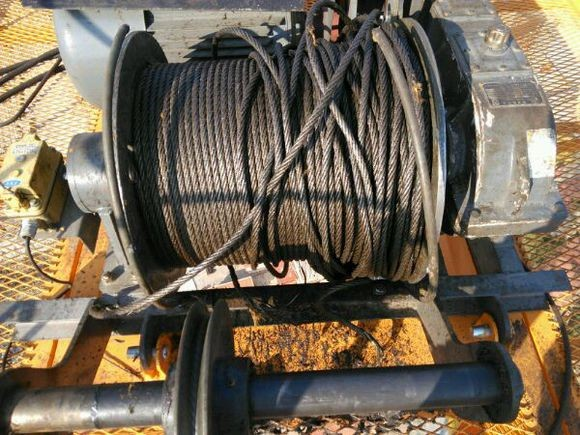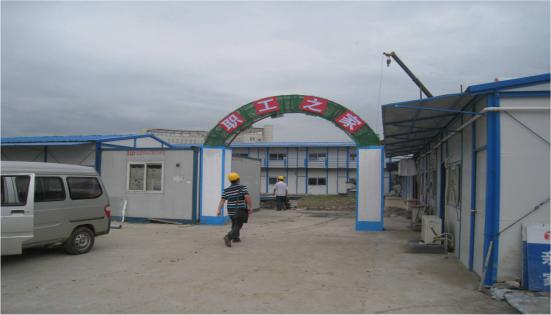# 2022年西藏拉萨建安b证在线模拟试题

[点击进入2022年西藏拉萨建安b证在线模拟试题]

A.一般事故

B.较大事故

C.重大事故

D.特别重大事故

A.四级(含四级)

B.五级(含五级)

C.六级(含六级)

D.七级(含七级)

A.一般危险源和特殊危险源

B.一般危险源和重大危险源

C.较小危险源和较大危险源

D.次要危险源和主要危险源

A.100

B.150

C.200

D.250

A.纵向刚度

B.竖向刚度

C.横向刚度

D.其他

A.20%

B.25%

C.30%

D.35%

A.防倾覆

B.防滚动

C.防滑移

D.防倾斜

A.100%

B.110%

C.120%

D.125%

A.龙门架式和井架式

B.上回转式和下回转式

C.高架和低架

D.行走式和固定式

A.设备

B.人群

C.水源

D.火源

A.谁主管，谁检查

B.谁用工，谁负责

C.谁负责

D.谁保管

A.10

B.15

C.20

D.25

A.25—40

B.30—50

C.40—65

D.45—65

A.1.5m

B.2m

C.1m

D.2.5m

A.城市管理部门

B.建设行政主管部门

C.公安部门

D.国土规划部门

A.没有要求

B.上下整齐

C.监理查验

D.安全验算

A.20日

B.30日

C.45日

D.60日

A.延退不报

B.退报

C.被举报

D.不报

A.30

B.40

C.60

D.120

A.燃气供应企业

B.使用者个人

C.燃气供应企业或个人

D.安全负责人

A.5

B.8

C.10

D.12

A.安全生产部门

B.安监局

C.安全生产委员会

D.应急管理局

A.分包单位

B.总包单位

C.建设单位

D.监理单位

A.半天

B.一天

C.两天

D.三天

A.总开关

B.急停开关

C.上极限开关

D.下极限开关

A.2

B.3

C.4

D.5

A.塔身的垂直度

B.起重臂的稳定性

C.平衡臂的稳定性

D.塔身的稳定性

A.1

B.2

C.3

D.5

A.设备租赁机构

B.检验检测机构

C.施工单位

D.建设单位

A.拟拆除建筑物.构筑物及可能危及毗邻建筑的说明

B.工程项目负责人.安全管理人员和特种作业人员持证上岗情况

C.拟用于安全生产和文明施工的措施费用清单

D.临时设施规划方案和已搭建情况

A.楼梯口.电梯井口.预留洞.通道口

B.电梯井口.预留洞.楼梯口.桥梁口

C.隧道口.电梯井口.预留洞口.通道口

D.孔洞口.电梯井口.隧道口.预留洞口

A.0.2

B.0.25

C.0.3

D.0.35

A.横向

B.纵向

C.四周

D.顶部

A.焊接设备

B.吊装机械

C.安装设施

D.大型机械

A.最大拉力

B.最小拉力

C.拉力

D.拉断力

A.1.8m

B.1.5cm

C.2.0cm

D.1.6cm

A.安全帽

B.安全带

C.安全网

D.安全标识A.力矩限制器

B.超高限制器

C.吊钩保险

D.钢丝绳防脱槽装置

A.不得在顶进过程中随意停机

B.不准无指挥开机

C.不得在顶铁两侧操作

D.不得盲目蛮干

A.建设单位.施工单位.监理单位

B.公司.项目.班组

C.建设单位.总包单位.分包单位

D.总包单位.分包单位.监理单位

A.剥裂

B.刻痕

C.锐角

D.接缝

E.裂纹

A.责令改正

B.没收违法所得，并处罚款

C.直接追究单位的刑事责任

D.可以责令停止整顿，降低资质等级

E.情节严重的，吊销资质证书

A.悬空高处作业

B.高处作业

C.露天作业

D.露天攀登

E.内装修作业

A.主要负责人

B.技术负责人

C.施工负责人

D.项目负责人

E.专职安全生产管理人员

A.连锁

B.隔离

C.减弱

D.预防

E.警告

A.标本兼治

B.综合治理

C.系统建设

D.齐抓共管

E.失职追责A.膳食

B.配件库

C.饮水

D.休息场所

E.危险物品库

A.封印损坏

B.超过校验有效期

C.表盘封面玻璃破裂

D.表盘刻度模糊不清

E.无压力时，指针距零位的数值没有超过压力表的允许误差

A.迟报

B.漏报

C.谎报

D.瞒报

E.通报

A.吊笼

B.物料

C.作业人员

D.其它

A.煤渣

B.煤灰

C.水泥

D.石灰

E.石膏

A.吊桩

B.回转

C.吊锤

D.行走

E.吊送桩器

A.建立安全生产责任制

B.安全设备.设施的更新维护和新技术.新工艺.新材料的应用

C.应急救援器材.设备

D.安全生产教育.培训.奖励费用

E.劳动保护设施.用品及职业危害防治费用

A.节能环保

B.防火间距

C.现场灭火

D.人员疏散

E.节约成本

A.临时消防设施是否完好有效

B.报警.接警处置的程序和通讯联系方式

C.临时消防车道及临时疏散设施是否畅通

D.应急灭火处置机构及各级人员应急处置职责

E.扑救初期火灾的程序和措施

A.安全防护装置应齐全.有效

B.根据使用情况选择配装二次侧漏电保护器

C.接地(接零)应良好

D.漏电保护器参数应匹配，安装应正确，动作应灵敏可靠

A.占用.堵塞.封闭消防安全出口.疏散通道和消防车通道

B.圈占.埋压.损坏.挪用.遮挡消防设施和器材

C.损坏公共消防设施

D.无照经营

E.无证驾驶

A.销售符合标准的非道路移动机械用燃料的

B.销售不符合质量标准的煤炭.石油焦的

C.生产.销售挥发性有机物含量不符合质量标准

D.销售不符合标准的机动车船燃料的

E.在禁燃区内销售高污染燃料的

A.施工单位资质等级证明

B.拟拆除建筑物.构筑物及可能危及毗邻建筑的说明

C.拆除施工组织方案

D.堆放.清除废弃物的措施硬京分料管责任

A.相互依存

B.相互制约

C.共同促进

D.相辅相成

E.此消彼长

A.正确

B.错误

A.正确

B.错误

A.正确

B.错误

A.正确

B.错误

A.正确

B.错误

A.正确

B.错误

A.正确

B.错误

A.正确

B.错误

A.正确

B.错误

A.正确

B.错误

A.正确

B.错误

A.正确

B.错误

A.正确

B.错误

A.正确

B.错误

A.正确

B.错误

A.正确

B.错误

A.正确

B.错误

A.正确

B.错误

A.正确

B.错误

A.正确

B.错误

A.正确

B.错误

A.正确

B.错误

A.正确

B.错误

A.正确

B.错误

A.正确

B.错误

A.正确

B.错误

A.正确

B.错误

A.正确

B.错误

A.正确

B.错误

A.正确

B.错误

A.正确

B.错误

A.正确

B.错误

A.正确

B.错误

A.正确

B.错误

A.正确

B.错误

A.正确

B.错误

A.正确

B.错误

A.正确

B.错误

A.正确

B.错误

A.正确

B.错误